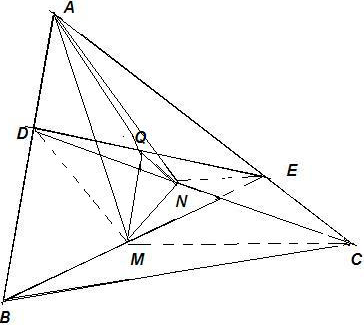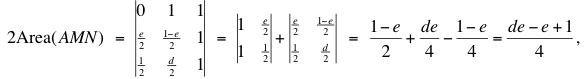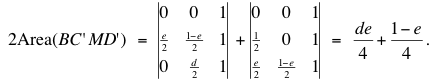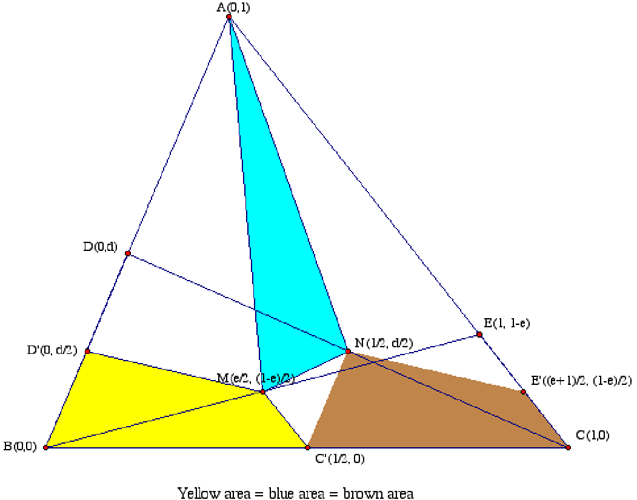SEARCH HOMEMath Central Quandaries & QueriesQuestion from Shamik, a student: ABC is any triangle and D and E are 2 points on AB and AC, respectively. M is the mid-point of BE and N is the mid-point of CD. Prove that the area of the triangle (AMN) is 1/4 of that of the quadrilateral(BCED). Use plane-geometry without using co-ordinates to find the solution. My Work Done: This can be achieved if I use analytical geometry with the help of co-ordinates. But, I started doing it by using 'plane geometry' tricks as specified in the problem. My work follow: A' ? midpoint of BC B' ? midpoint of CA C' ? midpoint of AB The locus of the point M is along A'C' as E moves from C to A. The locus of the point N is along A'B' as D moves from B to A. A'N = (1/2)*BD A'M = (1/2)*CE I thought a lot in the above lines but couldn't meet the solution. I have got an intuitive solution if I could attain the following. If I could consider one more mid-point somewhere in the diagram. If I could split the triangle(AMN) into parts such that it can be made equal to some quadrilateral which is 1/4 of DBCE. ThanksChris responded with a proof that uses coordinates and then Shamik produced his own proof. We have Shamik's proof first and then Chris'.

Please, refer to the figure. Here is the solution.Let Q be the midpoint of DE. Join QA, QM, QN, MD, MC and NE.

Triangle (AMN) = Triangle (AMQ) + Triangle (QMN) + Triangle (QNA)

Q, M and N are midpoints of DE, BE and CD, respectively. Therefore, QM is parallel to AB and QN is parallel to AC.

And, hence, Triangle (AQM) = Triangle (DQM) and Triangle (AQN) = Triangle (EQN)

So, Triangle (AMN) = Triangle (AQM) + Triangle (QMN) + Triangle (QNA)

= Triangle (DQM) + Triangle (QMN) + Triangle (EQN)

Thus, Triangle (AMN) = Quadrilateral (DMNE) ………………. (1)

M is the midpoint of BE and so Triangle (DME) = (1/2)* Triangle (BDE) while Triangle (CME) = (1/2)* Triangle (BCE)

è Triangle (DME) + Triangle (CME) = (½)*{Triangle (BDE) + Triangle (BCE))

From (1) and (2) we get, Triangle (AMN) = (1/4)*{Quadrilateral (DBCE)}

Shamik

Chris' proof

We simplified the problem a bit by first shrinking the quadrilateral BCED by ½: Take C' and D' the midpoints of BC and BD, then show that (AMN) = Area(BC'MD').  Using the coordinates shown in the figure, we computeandWas your argument this easy?  We will let you know if we find a simple argument that avoids coordinates.ChrisMath Central is supported by the University of Regina and The Pacific Institute for the Mathematical Sciences.